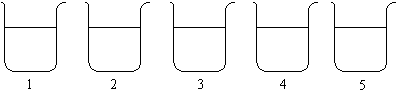## 22.4 The Iodine Clock or Landolt Reaction

Chemical Concepts Demonstrated: Kinetics, concentration effects on reaction rate

Demonstration:

 The 5 beakers contain 4 solutions in varying amounts: The solutions are poured into the beakers simultaneously. H2O Starch solution KIO3 solution Na2SO3 Beaker 5 contains the most amount of water and the least amount of KIO3 solution; Beaker 1 is the opposite.  The remaining beakers are in a series relative to these two.Observations:

The beakers turn blue-black in a specific order.

Explanation (including important chemical equations):

This demonstration is based on the following mechanism:

1. IO3-(aq) + 3 HSO3-(aq) --> I -(aq) + 3 SO42-(aq) + 3 H+(aq)

2. IO3-(aq) + 5 I -(aq) + 6 H+(aq) --> 3 I2 (aq) + 3 H2O (l)

3. I2 (aq) + HSO3-(aq) + H2O (l) --> 2 I -(aq) + SO42-(aq) + 3 H+(aq)

4. I2 (aq) +  I -(aq) + starch (aq) --> starch-I3-(aq)

Because steps 1 and 2 are much slower than step 3, no I2 accumulates as long as HSO3- remains in solution.   When all of the HSO3- is consumed, I2 accumulates and the blue-black starch-triiodide complex is formed in step 4.